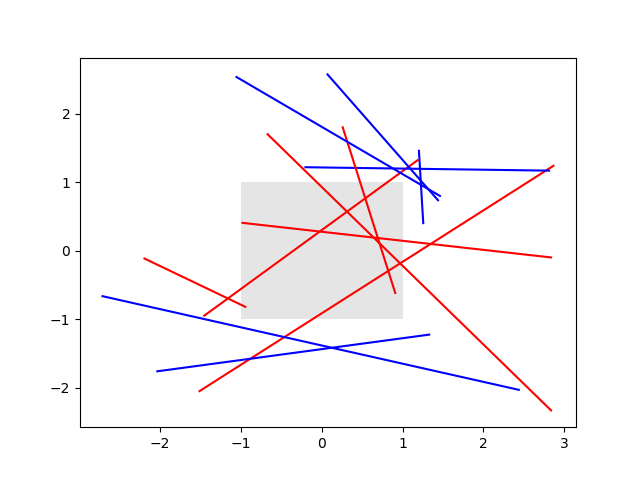# Changing colors of lines intersecting a box¶

The lines intersecting the rectangle are colored in red, while the others are left as blue lines. This example showcases the intersect_bbox function.import numpy as np
import matplotlib.pyplot as plt
from matplotlib.transforms import Bbox
from matplotlib.path import Path

# Fixing random state for reproducibility
np.random.seed(19680801)

left, bottom, width, height = (-1, -1, 2, 2)
rect = plt.Rectangle((left, bottom), width, height,
facecolor="black", alpha=0.1)

fig, ax = plt.subplots()

bbox = Bbox.from_bounds(left, bottom, width, height)

for i in range(12):
vertices = (np.random.random((2, 2)) - 0.5) * 6.0
path = Path(vertices)
if path.intersects_bbox(bbox):
color = 'r'
else:
color = 'b'
ax.plot(vertices[:, 0], vertices[:, 1], color=color)

plt.show()


Keywords: matplotlib code example, codex, python plot, pyplot Gallery generated by Sphinx-Gallery